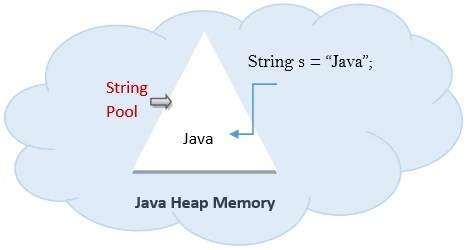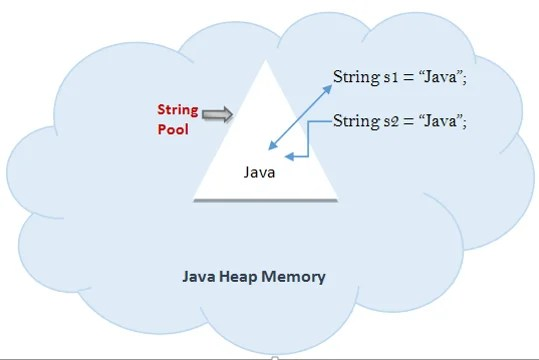Home » Java Tutorial » Explore String in Java

# Exploring String class in Java

In Java, String class is a sub-class of java.lang and there are available to all programs automatically. Java implements String as objects of String unlike other programming language which implements it as character arrays.

### String Constructors:

1. The string class can be initialized using several constructors. To create an empty string, default constructor is as follows:

Above will create an instance of String with no characters in it.

1. To create a String containing character sequence use below constructor:

`String(char chars[ ])`

For example:

`char chars[] = { 'a', 'b', 'c' };`

String s = new String(chars);

In the above example a string object will be created having ‘abc’ as its value.

1. String object can also be passed as constructor argument.

`String(String strObj)`

See below example:

`class MakeString {`

`public static void main(String args[]) {`

`char c[] = {'J', 'a', 'v', 'a'};`

`String s1 = new String(c);`

`String s2 = new String(s1);`

`System.out.println(s1);`

`System.out.println(s2);`

`}`

`}`

The output from this program is as follows:

`Java`

`Java`

As you can see, s1 and s2 contain the same string.

1. String class provides constructors that initialize a string when given a byte array.

`String(byte asciiChars[ ])`

`class SubStringCons {`

`public static void main(String args[]) {`

`byte ascii[] = {65, 66, 67, 68, 69, 70 };`

`String s1 = new String(ascii);`

`System.out.println(s1);`

`}`

`}`

This program generates the following output:

`ABCDEF`

1. String can also be initialized using below code as well:

`String s = new String(“JAVA”);`

### String Length:

The string length is the number of characters in contains. This value can be obtained using length() method. Its return type is int.

`String s = new String(“JAVA”);`

`System.out.println(s.length());`

The above will print 4 because there are 4 characters in the string “JAVA”.

### String Literals:

We saw that how a string can be constructed through array of characters using new keyword. Java provides simpler way to construct a string using literals. For each string literal in the program Java automatically constructs a string object.

`String s = “Java”;`

The above will create a string object and it can be used all the string operations and manipulations in the program. Like below line of code will print string length as 4.

`System.out.println(s.length());`

Difference between initializing a string by literal and by new keyword:

Before going into the details let’s understand the Java Heap and String Pool. Those are basically memory spaces that JVM keeps with itself since its startup. Before Java 1.7 these are two separate memory allocations but from Java 1.7 onwards String pool occupies some space from Java Heap itself. Please see the below diagram.String Pool is a memory pool inside Java heap where String value created by literal will be placed out.

`String s = "Java";`

When above code will be executed then it will look into the String pool for the same value. If it is found then s will refer to that but if it is not found then a new String with value (Java as in case) will be created and s will refer to this value. So if we will create another string in the pool with the same value like below:

`String s1 = "Java";`

It will not create any new string in the pool but will refer to the string created by previous code.

Consider below lines of code:

`String s = "abodeqa";`

`String s1 = "abodeqa";`

`System.out.println( s==s1 );`

Above will print true because both the string objects are referring to the same memory location. But when string object is created using new keyword:

`String s1 = new String ("Java");`

then string object will be created in the Java Heap but not in string pool.also if another string object is created using new keyword having same value it will create another fresh object in Java heap unlike the literal. See above diagram.

`String s1 = new String("abodeqa");`

`String s2 = new String("abodeqa");`

`String s3 = "abodeqa";`

`System.out.println( s1==s2 ); // return false because both the object are referring to different memory locations`

`System.out.println( s1==s3 ); // return false because s1 is in heap and s3 is in string pool`

`System.out.println( s1.equalsTo ); // return true`

We can use intern() method on the string created by new keyword. This will create the string in the string pool.

`String s = "Java";`

`String s1 = new String("Java");`

`String s2 = new String("Java").intern();`

`System.out.println(s==s2); // true`

`System.out.println(s==s1); //false`

`System.out.println(s1==s2); //false`I hope above explanation is understandable. If you have doubts please comment on the same. It will be answered to the best of my knowledge.